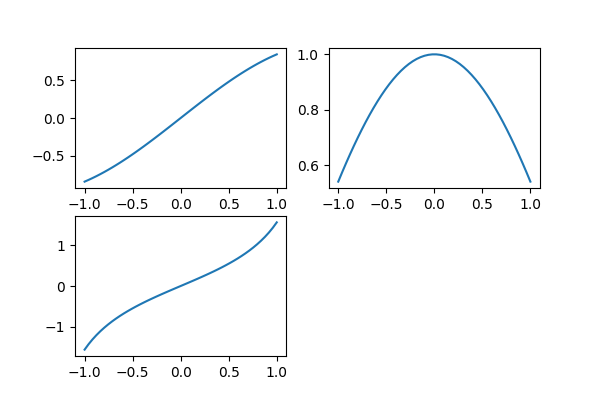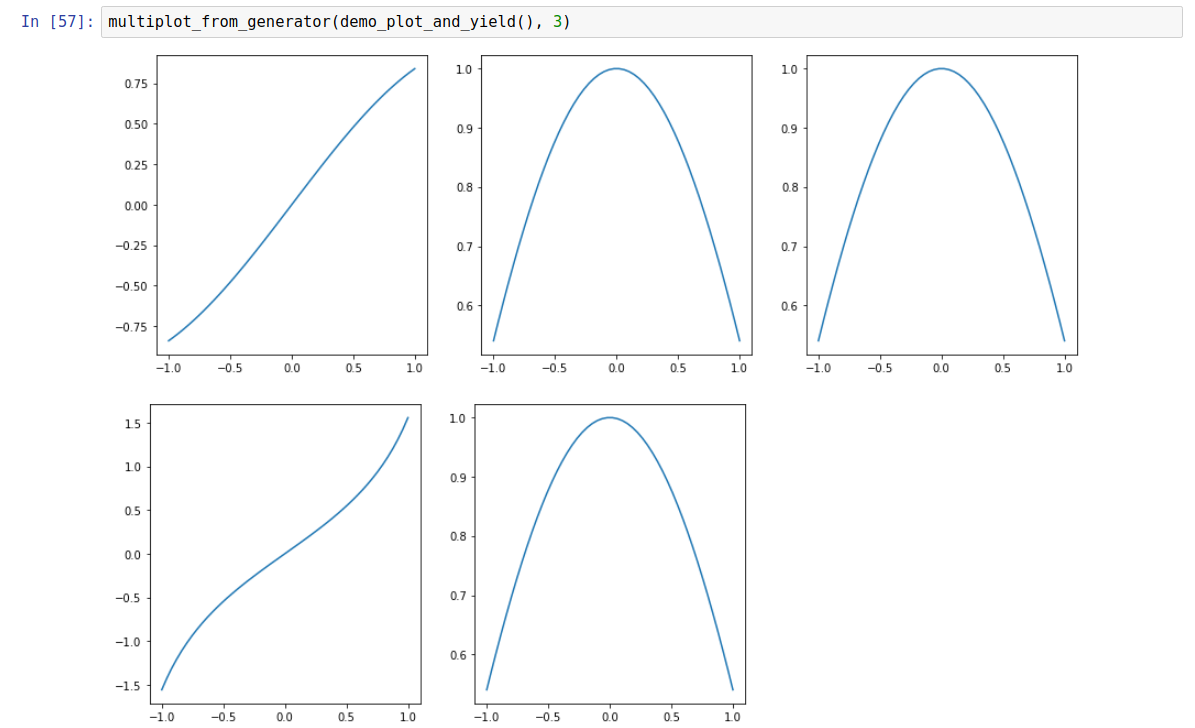# Aaron McDaid

Statistics and Python and C++, and other fun projects

aaron.mcdaid@gmail.com

@aaronmcdaid

by Aaron, 2nd January 2019

(code for this document)

When searching online for how to do multiple plots in a Python Jupyter notebook, you often get are told to write code like this (“Multiple subplots”):

import matplotlib
import numpy as np
import matplotlib.pyplot as plt
xs = np.linspace(-1, 1)
plt.subplot(2,2,1)
plt.plot(xs, np.sin(xs))
plt.subplot(2,2,2)
plt.plot(xs, np.cos(xs))
plt.subplot(2,2,3)
plt.plot(xs, np.tan(xs))
plt.show()This works well. However, I find this difficult for a number of reasons. It requires you know the number of items in advance and it is necessary to manually keep track of the coordinates, making the correct call to subplot each time. Also, it mixes up your plotting code with this subplot-management code.

So instead, here is a simple trick to allow you to specify all your plots, separated by yield:

def demo_plot_and_yield():
xs = np.linspace(-1, 1)
# Put a 'yield' before every subplot, including the first one:
yield
plt.plot(xs, np.sin(xs))
yield
plt.plot(xs, np.cos(xs))
yield
plt.plot(xs, np.cos(xs))
yield
plt.plot(xs, np.tan(xs))
yield
plt.plot(xs, np.cos(xs))

Note: There must be a yield before every plot, including the first one.

Then you can send that function to multiplot_from_generator (defined below) along with the desired number of columns (in this case 3):

multiplot_from_generator(demo_plot_and_yield(), 3)

Here is the result in Jupyter:optional caption text

## How to enable it

You simply need to define the multiplot_from_generator function as follows. Note the figsize parameter, which you might override if you don’t like the default. By default, the width of one row is 15 “inches” as this seems to be the width of a Jupyter notebook. Also, by default the subplots are square; we do this by setting the height to 15/num_columns:

def multiplot_from_generator(g, num_columns, figsize_for_one_row=None):
# call 'next(g)' to get past the first 'yield'
next(g)
# default to 15-inch rows, with square subplots
if figsize_for_one_row is None:
figsize_for_one_row = (15, 15/num_columns)
try:
while True:
# call plt.figure once per row
plt.figure(figsize=figsize_for_one_row)
for col in range(num_columns):
ax = plt.subplot(1, num_columns, col+1)
next(g)
except StopIteration:
pass

## How it works

multiplot_from_generator sets up the rows and columns in a loop and then calls next(g) each time in order to force the next piece of plotting code in the generator to be executed.

next(g) causes the generator to run until the next yield.

I did try a different design where the yield was required after (not before) each subplot. But that ran into difficulties where I needed to delete the last subplot. So this design, with the yield in front` works better.

Thanks for making it this far! I’d love feedback, see my Twitter handle and my email address at the top of the page.

by Aaron, 2nd January 2019